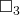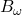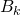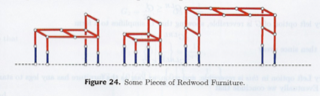# Tag Archives: Difficulty 8

## Conjunctive Satisfiability with Functions and Inequalities

The problem: Conjunctive Satisfiability with Functions and Inequalities.  This is problem LO16 in the appendix.

The description: Given a set U of variables, a set F of functions on 1 variable, a collection C of clauses connecting 2 statements U and V with either:

• >
• =

.. and U and V are either

• A constant 0 or 1
• f(0) or f(1) for some f in F
• f(u) for some u in U, and some f in F

.. can we find integer values to all variables in U that make all of the clauses in C true?

Example:

Let F = {f,g}, f(x) = x2, g(x) = x3.  Let U = {a,b}

Let our clauses be:

• f(a) > g(b)
• a ≤ b

We can satisfy the clauses by choosing a = -2 and b = 1.  If we had another clause that said a > 2 then the clauses would not be satisfiable.

Reduction: G&J point you to an “unpublished manuscript” by Pratt, which I found online.  Some people who reference this paper just call it a “manuscript” and some people call it a Tech Report from MIT.  I looked at the MIT tech report list and couldn’t find this.  But that doesn’t really mean anything since not all of the tech reports from the 1970s have been indexed online at a lot of places.  Anyway, you can find the paper online.

I do wish the paper had gone through an editing pass because it has what to me look like bugs or ambiguities.  Maybe I’m not understanding things, though.

Here’s the basic idea: Given a Satisfiability formula P, build a formula H(P) in this new construction.  The clauses are:

• Each variable has to be 0 or 1 (so, 0 ≤ u and u ≤1 for all u in U)
•  Each literal i has a distinct function fi.  If the literal is positive then we will be using fi(0) in what’s to come.  If the literal is negative then we will be using fi(1) in what’s to come.
• If 2 literals P and Q are connected by ∧, create clauses for the inequalities:
• fp(0 or 1) ≤ fq (0 or 1)  (0 or 1 depending on whether the literal is positive or negative
• fp(P) ≤ fq(Q)
• If 2 literals P and Q are connected by ∨ (the paper uses the | symbol for “or” which I’d never seen before), create a clause for the inequalities:
• fp(P) ≤ fq (0 or 1)
• f0 (0 or 1) > fP(P) for some(?) (maybe all?) variable P.

In the description, Pratt says that they’re building a graph to represent the formula connected by inequalities.  If we hit a ∧ symbol, it will connect the graphs of the two parts of the formula together with a parallel connection, so that there is always a way to get from one subgraph to another.  If we hit a ∨ symbol, the subgraphs are connected by a series connection.  He says “We hope that this informal discussion will make a more formal argument unnecessary”.  I for one wish there was some more detail here.

The examples he uses to explain what’s happening are just on the trivial formulas “a” and “a ∧ ~a”.  I can sort of see how the clauses he creates come out of those formulas.  But since none of his examples use the ∨ operation, or use more complex clauses, it’s hard for me to see what happens there.  I’m probably missing something.

It also seems like he wants to let the definition of the functions be part of the satisfiability argument (i.e. not only do we have to find truth values for all of he variables, we also have to create functions that make all of the clauses become true), which seems to not be the definition provided in G&J.

Difficulty: 8.  There is probably a much simpler reduction out there.  I wish I knew what it was.

## Modal Logic Provability

Here’s a much harder reduction based on the modal logic from last time.

The problem: Modal Logic Provability.  This is problem LO14 in the appendix.

The description: Given a modal system S that follows the “S4” modal logic rules, and a modal statement A, can A be proven in S?

Example: S4 modal logic is concerned with what different agents know.  I found a pretty good example of how it works from the notes of a lecture at CMU (Section 2, the story about the hats).

From our point of view, we would say that statement A would beB3. (The third person knows his hat is black), and the explanation of how we got there would be the proof of the statement.

Reduction: Ladner’s paper has the reduction from QBF.  It’s theorem 3.1 in the paper if you want to look at it.  They start with the setwhich is the set ofsets that the Stockmeyer and Meyer paper used in their QBF proof (I talked about it in my discussion of Sequential Truth Assignment), but with any number of quantifiers.  From a QBF formula A, they will build a modal formula B (sort of like the A in our problem definition) with the property that A is a satisfiable QBF formula if and only if B is S4-Satisfiable.

(He actually proves that it for B  “between” K-Satisfiable and S4-Satisfiable.  K-satisfiable is a slightly simpler set of rules.)

The way he builds B is pretty crazy and hard to follow.  I’m not sure there’s much I can say about it that’s not just requoting the entire paper.  The basic idea though is that he is using the modal operators in B to replace the quantifiers in the QBF formula.  The various levels of “for all” and “there exists” are replaced by worlds for agents “know” that a fact is true.

So the clauses in the formula form a tree, and every “For all” in A leads to a branch of worlds (one where the variable is true, and one where it is false).  Then he shows that

Difficulty:8.  Maybe less if you’re comfortable with the Modal logic rules.

## Generalized Instant Insanity

The last problem in the “Games and Puzzles” section comes from a commercial puzzle (though one based on a much older puzzle).  It’s cool how these sorts of things pop up- a toy or game comes out and then people analyze it mathematically and find some neat features about it.

The problem: Generalized Instant Insanity.  This is problem GP15 in the appendix.

The description: Given a set Q of cubes, and a set C of colors we paint on the sides of each cube (and where |Q| = |C|) is there a way to arrange the cubes in a stack such that each color in C appears exactly once on each side?

Example: The Wikipedia page for the Instant Insanity game has a good description of the game and an example of the solution when Q = 4.

Reduction: Roberson and Munro reduce from “Exact Cover”.  I don’t think I’ve done this problem, but it’s basically X3C where the sizes of the sets can be any size.  (So it’s a trivial reduction from X3C).

They start by designing a graph representation of the puzzle, similar to the one shown on the Wikipedia page. Vertices correspond to colors, an edge connects two vertices if they are on opposite sides of the came cube, and the edges are labeled with the name of the cube.  The puzzle has a solution if and only if we can find two edge disjoint cycles that touch each vertex and edge label exactly once.  The two cycles correspond to the two sets of opposite faces we can see (since 2 sides of each cube are hidden by the stack).  Again, go look at the graphs in the Wikipedia article– they show this pretty nicely.

So, to perform the reduction, we’re given a set S {s1..sm} and a collection T of subsets of S, and need to make a graph (which corresponds to a puzzle).  Each element in S will become a vertex in the graph.  Each of these vertices will have a self-loop and be labeled ζi (So each vertex is a different color, and each self-loop is part of a different cube- so each cube has one color on opposite sides).

Each element si inside a set Th of T will also have a vertex: vi,h. This vertex has an edge with the next largest level sj within Th, wrapping around if we’re at the last element of Th. These edges are labeled ϒh,j.  We also have an edge from sjto vh,j if sj is in Th labeled with δh,j.  We also have 2 copies of self-loops from each vh,j to itself labeled ϒh,j.

They then add some extra edges to give the graph the properties:

• One of the self-loops from vh,i to itself has to be in one of the two cycle sets.
• If the edge from vh,i to sj (labeled ϒh,j) is in the other cycle set, then the edge from sj to vh,j (labeled δh,j) is also in that cycle set
• The ζi edge label has to be used, and so will have to be used on an si vertex the cycle that uses that vertex will also have to have the labels ϒh,j and δh,j for some h.
• The loops (labeled ζj and ϒh,j) are in one cycle set and the paths (labeled ϒh,j and δh,j) are in the other.

With these properties, then solving the puzzle means we have to find h1 through hm that correspond to sets Th in T, but also where there is a path ϒh,jh,j in the graph for each sj in Th_i.  We make sure no elements in S are repeated by making sure the puzzle only uses each sj vertex once.

Difficulty: 8.  I glossed over a lot of the construction, especially the definition of a “p-selector”, which is a subgraph that helps set up a lot of the properties above.  That definition is very hard for me to follow.

## Left-Right Hackenbush for Redwood Furniture

Here’s an interesting problem, but a hard one to explain.  Most of what I’m doing here comes from the very cool “Winning Ways for Your Mathematical Plays” book by Berlekamp, Conway, and Guy, which I hope at some point in the future to have the time to really dig deeply into.  But for now, I’ll just use it as a reference to this week’s problem.

The problem: Left-Right Hackenbush for Redwood Furniture.  This is problem GP12 in the appendix.

The description: Ok, here we go.  First, a Hackenbush problem consists of an undirected, connected graph.  The edges of the graph are marked as “Left” or “Right”(though the book has some very nice colored pictures, where the edges are labeled “Blue” and “Red”).  Some of the vertices are on the ground (In G&J’s definition, there is one ground vertex, but it’s equivalent to having several vertices that are all on the ground).

On Left’s turn, they remove a blue edge and then all edges that are not connected to the ground are removed.  On Right’s turn, they remove a red edge, and then all edges that are not connected to the ground are removed.  A player loses if there are no remaining edges of their color.

redwood furniture Hackenbush instance is one where:

• No red edges touch the ground
• Each blue edge (or “foot”) has one end on the ground and the other touches a unique red edge (the “leg”)

Here are some redwood furniture instances from the book:The “value” of a Hackenbush position is the number of “spare” moves (with optimal play) one player has after the other player loses.  A value of 0 means that whoever’s turn it is will lose (on an empty board).  The definition can be extended to fractional values.  For example, a value of 1/2 for (say) Left means that if we made two copies of the game, we would end up with a situation with a value of 1 for Left.

So, the question is, for some Redwood Furniture graph, and some K, is the value <= 2-K?

Reduction:

I’m just going to sketch the process here since it takes several pages of the book (and depends on results and ideas from earlier in the book).

They show:

• The value of any redwood furniture graph is 2-N for some N.  In the degenerate case, the graph with just one Left edge has a value of 1. (=20)
• On Left’s turn, they will always remove a foot (by definition, that’s all they have).  On Right’s turn, they should make a move that does not disconnect the graph, if possible.
• A “Bed” is a redwood furniture graph where every red edge that is not a leg connects to a leg.  It has value 2-(m+1), where m is the largest number of moves that do not disconnect the bed.
• The value of the game depends on how many extra moves Red has to get down to just a bed.
• To find m, you need to know the size of the largest redwood tree (a tree is a graph that will be disconnected by the removal of any edge) that contains all of the legs.
• The edges of the bed (the red edges that are not legs) form a bipartite graph.  So finding m is equivalent to the set covering problem, where the elements of the set are the vertices, and the edges are the sets.

Here’s how I think the Set Covering reduction works.  Given a set covering instance: a set S of elements, and a collection C of subsets of S, we’ll build a bipartite graph.  One set of the bipartite graph will correspond to the elements of S (and will be on the legs of the furniture graph).  The other set will correspond to the elements in C (and will be the vertices in the bed that are not in the legs).  An edge will go from each “C vertex” to each “S vertex” in its set.  Now, the cover is the set of vertices from the bed that cover all of the legs.

The book says you want the “smallest redwood tree which contains all of the legs”, which I think is the same thing (smallest number of extra vertices), but I’m not 100% confident since the Hackenbush game involves removing edges, and we’re choosing vertices in the cover.

I’m a little sad that the book does such a great job describing the game, and the value function, and then glosses over the reduction part (and uses misleading terms like “Minimum Spanning Tree of a Bipartite Graph”, which is a polynomial problem).  The actual reduction in G&J is to a private communication, so that’s not much help.

Difficulty: Boy, I don’t know, I think it depends on where you start from.  If my Set Cover reduction is the right one, and all you ask a student to do is that, it’s probably a 4.  If you’re going to make them prove all of the things I glossed over about the value number, then it probably goes up to at least an 8.